• +91 9971497814
• info@interviewmaterial.com

# Arithmetic Progressions Ex-5.3 Interview Questions Answers

### Related Subjects

Question 1 : Find the sum of the following APs.

(i) 2, 7, 12 ,…., to10 terms.
(ii) − 37, − 33, − 29 ,…, to 12 terms
(iii) 0.6, 1.7, 2.8 ,…….., to 100 terms
(iv) 1/15, 1/12, 1/10, …… , to 11 terms

Solution

(i) Given, 2, 7, 12 ,…, to 10 terms

For this A.P.,

first term, a = 2

And common difference, d = a2 − a1 =7−2 = 5

n = 10

We know that, the formula for sum of nth termin AP series is,

Sn = n/2 [2a +(n-1)d]

S10 =10/2 [2(2)+(10 -1)×5]

= 5[4+(9)×(5)]

= 5 × 49 = 245

Solution

(ii) Given, −37, −33, −29 ,…, to 12 terms

For this A.P.,

first term, a = −37

And common difference, d = a2− a1

d= (−33)−(−37)

= − 33 + 37 = 4

n = 12

We know that, the formula for sum of nth termin AP series is,

Sn = n/2 [2a+(n-1)d]

S12 =12/2 [2(-37)+(12-1)×4]

= 6[-74+11×4]

= 6[-74+44]

= 6(-30) = -180

Solution

(iii) Given, 0.6, 1.7, 2.8 ,…, to 100 terms

For this A.P.,

first term, a = 0.6

Common difference, d = a2 − a1 =1.7 − 0.6 = 1.1

n = 100

We know that, the formula for sum of nth termin AP series is,

Sn = n/2[2a +(n-1)d]

S12 = 50/2 [1.2+(99)×1.1]

= 50[1.2+108.9]

= 50[110.1]

= 5505

Solution

(iv) Given, 1/15, 1/12, 1/10, …… , to 11 terms

For this A.P.,

First term, a = 1/5

Common difference, d = a–a1 =(1/12)-(1/5) = 1/60

And number of terms n = 11

We know that, the formula for sum of nth termin AP series is,

Sn = n/2 [2a + (n –1) d]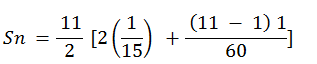= 11/2(2/15 + 10/60)

= 11/2 (9/30)

= 33/20

Question 2 :

Find the sums givenbelow:

Answer 2 :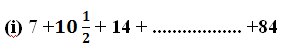(ii) 34 + 32 + 30 +……….. + 10
(iii) − 5 + (− 8) + (− 11) + ………… + (− 230)

Solution
(i)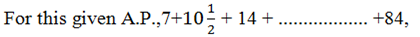First term, a = 7

nth term, a=84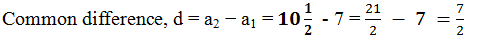Let 84 be the nth termof this A.P., then as per the nth term formula,

a= a(n-1)d

84 = 7+(n – 1)×7/2

77 = (n-1)×7/2

22 = n−1

n = 23

We know that, sum of n term is;

Sn = n/2(a + l) , l = 84
Sn
= 23/2 (7+84)

Sn  = (23×91/2) = 2093/2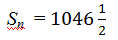Solution

(ii) Given, 34 + 32 + 30 + ……….. + 10

For this A.P.,

first term, a = 34

common difference, d = a2−a1 =32−34 = −2

nth term, an= 10

Let 10 be the nth termof this A.P., therefore,

an= a +(n−1)d

10 = 34+(n−1)(−2)

−24 = (n −1)(−2)

12 = n −1

n = 13

We know that, sum of n terms is;

Sn = n/2 (a +l) , l= 10

= 13/2 (34 + 10)

= (13×44/2) = 13 × 22

= 286

Solution

(iii) Given, (−5) + (−8) + (−11) + ………… + (−230)

For this A.P.,

First term, a = −5

nth term, an= −230

Common difference, d = a2−a1 =(−8)−(−5)

d = − 8+5 = −3

Let −230 be the nth termof this A.P., and by the nth term formula we know,

ana+(n−1)d

−230 = − 5+(n−1)(−3)

−225 = (n−1)(−3)

(n−1) = 75

n = 76

And, Sum of n term,

Sn = n/2 (a + l)

= 76/2 [(-5) + (-230)]

= 38(-235)

= -8930

Question 3 : In an AP

(i) Given a =5, d = 3, an = 50, find n and Sn.
(ii) Given a = 7, a13 = 35,find d and S13.
(iii) Given a12 = 37, d = 3,find a and S12.
(iv) Given a3 = 15, S10 =125, find d and a10.
(v) Given d = 5, S9 = 75,find a and a9.
(vi) Given a = 2, d = 8, Sn =90, find n and an.
(vii) Given a = 8, an = 62, Sn =210, find n and d.
(viii) Given an = 4, d = 2, Sn =− 14, find n and a.
(ix) Given a = 3, n = 8, S =192, find d.
(x) Given l = 28, S = 144 and there are total9 terms. Find a.

Solution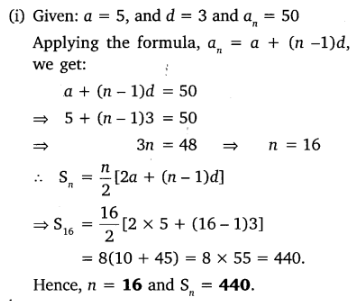Solution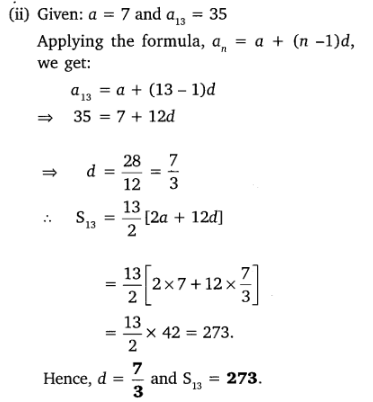Solution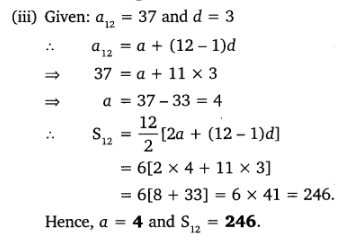Solution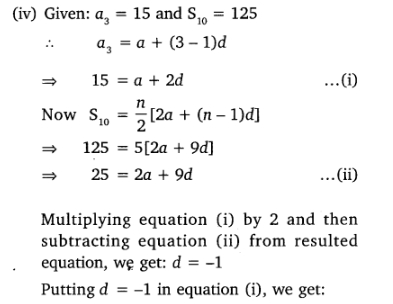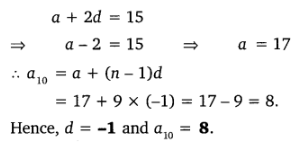Solution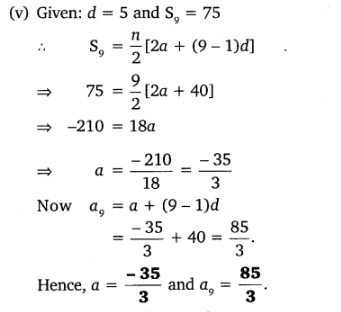Solution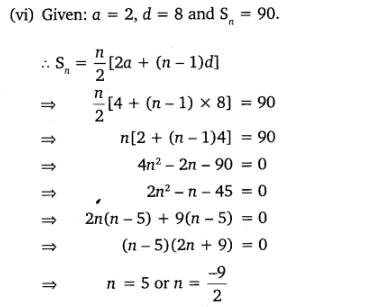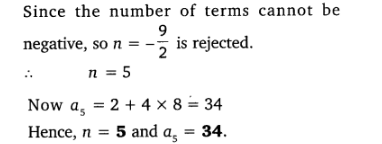Solution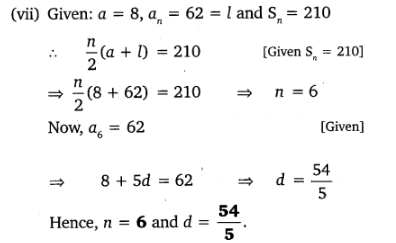Solution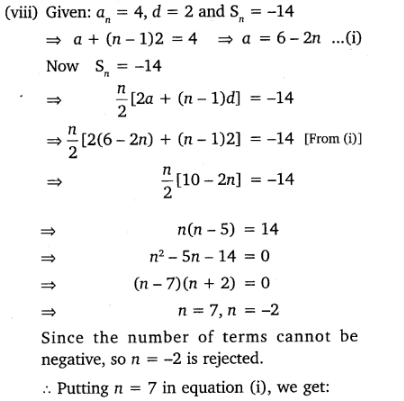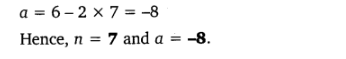Solution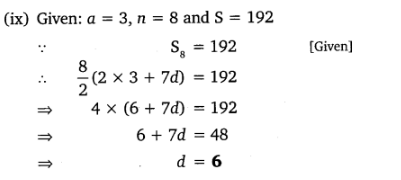Solution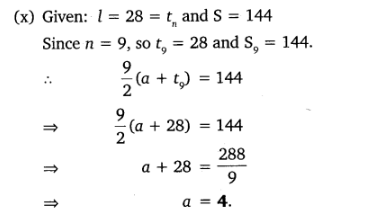Question 4 : How many terms of AP: 9, 17, 25, … must be taken to give a sum of 636?

Answer 4 :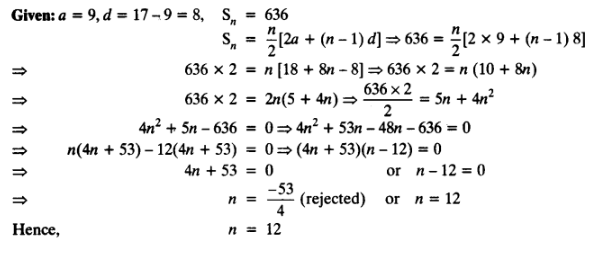Question 5 : The first term of an AP is 5, the last term is 45 and the sum is 400. Find the number of terms and the common difference.

Answer 5 :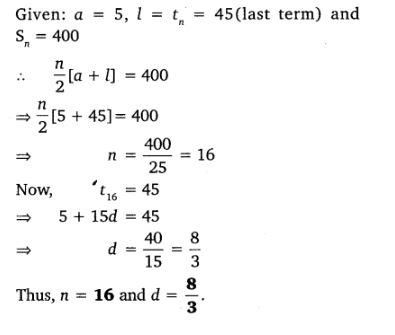Question 6 : The first and the last terms of an AP are 17 and 350 respectively. If the common difference is 9, how many terms are there and what is their sum?